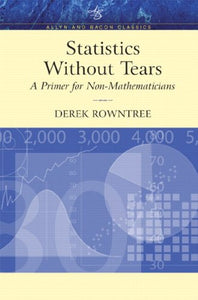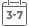# Statistics Without Tears: A Primer For Non-Mathematicians (Allyn & Bacon Classics Edition)

Vendor
Pearson
Regular price
\$95.31
Sale price
\$35.02
Quantity must be 1 or more

Attention: For textbook, access codes and supplements are not guaranteed with used items.This classic book uses words and diagrams, rather than formulas and equations, to help readers understand what statistics is, and how to think statistically. It focuses on the ideas behind statistics only; readers are not required to perform any calculations.

CONTENTS of STATISTICS WITHOUT TEARS

Introduction. How to use this book.

1. Statistical inquiry. Making sense of experience. What is statistics? Descriptive and inferential statistics. Collecting a sample.

2. Describing our sample. Statistical variables. Error, accuracy and approximations.

3. Summarizing our data. Tables and diagrams. Central tendency (averages). Measures of dispersion.

4. The shape of a distribution. Skewed distributions. Introducing the normal distribution. Proportions under the normal curve. Comparing values.

5. From sample to population. Estimates and inferences. The logic of sampling. A distribution of sample-means. Estimating the population-mean. Estimating other parameters.

6. Comparing samples. From the same or different populations? Significance testing. The significance of significance. Comparing dispersions. Non-parametric methods.

7. Further matters of significance. One- versus two-tailed tests. z-tests and t-tests. Comparing several means. Comparing proportions.

8. Analysing relationships. Paired values. Three kinds of correlation. The strength of a correlation. The significance of a correlation coefficient. Interpreting a correlation coefficient. Prediction and regression.

Postscript. Review. Caution.

Bibliography.

Index.